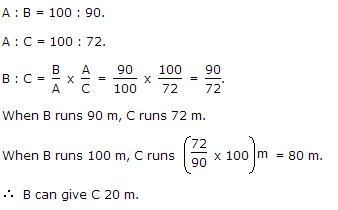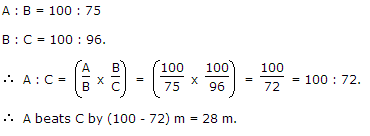# Races and Games Questions

FACTS  AND  FORMULAE  FOR  RACES  AND  GAMES  QUESTIONS

1. Races : A contest of speed in running, riding, driving, sailing or rowing is called a race.

Race Course : The ground or path on which contents are made is called a race course.

Starting Point : The point from which a race beigns is known as a starting point.

Winning Point or Goal : The point set to bound a race is called a winning point or a Goal.

Winner : The person who first reaches the winning point is called a winner.

Dead Heat Race : If all the persons contesting a race reach the goal exactly at the same time, then the race is said to be a dead heat race.

Start : Suppose A and B are two contestants in a race. If before the start of the race, A is at  the starting point and B is ahead of A by 12 metres, then we say that 'A gives B, a start of 12 metres'.

To cover, a race of 100 metres in this case, A will have to cover 100 metres while B will have to cover only (100 - 12) = 88 metres.

In a 100m race, 'A can give B 12m' or 'A can give B a start of 12m' or 'A beats B by 12m' means that while A runs 100m, B runs (100-12)=88m

2. Games : A game of 100, means that the person among the contestants who scores 100 points first is the winner?

If A scores 100 points while B scores only 80 points, then we say that 'A can give B 20 points'.

Q:

In a 100 m race, A beats B by 10 m and C by 13 m. In a race of 180 m, B will beat C by:

 A) 5.4m B) 4.5m C) 5m D) 6m

Explanation:

A : B = 100 : 90.

A : C = 100 : 87.

B/C = (B/A*A/C) = (90/100*100/87) = 30/29

When B runs 30 m, C runs 29 m.

When B runs 180 m, C runs (29/30*180)m =174m

B beats C by (180 - 174) m = 6 m.

118 103919
Q:

In a 1 km race, A beats B by 28 meters in 7sec. Find A's time over the course?

 A) 5min,4sec B) 4min,3sec C) 2min,3sec D) 3min,4sec

Explanation:

B covers 28 meters in 7sec. So, B's time over the course = (7/28)*100 =250 sec.

Whereas A's time over the course = 250 -7 = 243 sec.

i.e A's time over the course is 4min , 3 sec.

93 56593
Q:

In a 100 m race, A can give B 10 m and C 28 m. In the same race B can give C

 A) 18 m B) 20 m C) 27 m D) 9m

Explanation:42 50534
Q:

In a 100 m race, A can beat B by 25 m and B can beat C by 4 m. In the same race, A can beat C by

 A) 21 m B) 26 m C) 28m D) 29m

Explanation:50 49665
Q:

A and B take part in 100 m race. A runs at 5 kmph. A gives B a start of 8 m and still beats him by 8 seconds. The speed of B is:

 A) 5.15 kmph B) 4.14 kmph C) 4.25 kmph D) 4.4 kmph

Explanation:

A's speed = (5*5/15)m/sec  = (25/18)m/sec

Time taken by A to cover 100 m = (100*18/15)sec = 72sec

Time taken by B to cover 92 m = (72 + 8) = 80 sec.

B's speed =(92/80*18/5)kmph =4.14kmph

52 45223
Q:

In a 500 m race, the ratio of the speeds of two contestants A and B is 3 : 4. A has a start of 140 m. Then, A wins by:

 A) 60m B) 40m C) 20m D) 10m

Explanation:

To reach the winning post A will have to cover a distance of (500 - 140)m, i.e., 360 m.

While A covers 3 m, B covers 4 m.

While A covers 360 m, B covers (4/3)*360 = 480 m.

Thus, when A reaches the winning post, B covers 480 m and therefore remains 20 m behind.

A wins by 20 m.

42 44056
Q:

In 100 m race, A covers the distance in 36 seconds and B in 45 seconds. In this race A beats B by:

 A) 20m B) 25m C) 22.5m D) 9m

Explanation:

Distance covered by B in 9 sec. = (100/45)*9m = 20m

A beats B by 20 metres.

58 41491
Q:

A can run 22.5 m while B runs 25 m. In a kilometre race B beats A by:

 A) 100 m B) 75 m C) 25 m D) 50m

Explanation:

When B runs 25 m, A runs 45/2 m

When B runs 1000 m, A runs (45/2*1/25*1000)m = 900 m.

B beats A by 100 m.